# Sneak view into TensorFlow library and simple implementation of NAND

Introduction to TensorFlow :- TensorFlow is a library which is used by another library known as Keras. TensorFlow sits on the top of hardware (i.e. the machine where the program is being executed/ran). Machine can be as simple as our local workstation i.e. CPU as well as, it can be as good as GPU. We, the users of the system, As Developers works closely with Keras, but we can directly access to TensorFlow as well.

• It is an open-source library for numerical computation and is developed by Google.
• It can run across multiple GPUs or CPUs across several servers.
• If we have to make any compute-type of instruction as executable, then we can do it in form a graph. Same is very well supported by Tensorflows too.
• The power of TensorFlows is such that, it can perform the mathematical computations by using the parrallelizibility. TensorFlow works like a parallel processing unit, into which lot of data can be pumped-in.
• We can run a Single Instruction Steam. It’s based on Single Instruction, Multiple Data based pattern.

Let’s now first import the basic libraries which we shall be using in this blog. Please note here that, we shall be demonstrating the behaviour of TF library V1 and therefore we have explicitly disabled the behaviour of TF 2.0.

Next, let’s declare the two variables with name as : x and y. Here, we have initialised the values of x and y as 4 & 5 respectively. Also let’s declare a function : f(x,y) : which shall be using both of these declared variables along with initialisation.

Let’s now initialise all the global variables using method ‘global_variables_initializer()’.

Next, we would initialise a TensorFlow Session object using “tf.Session”, which would encapsulate the environment in which :-

• Init is being ran.
• Operation on objects are executed i.e. function is evaluated.
• Tensor objects are evaluated i.e. results of mathematical computation are obtained.

Next, we can also obtain the corresponding graph for this mathematical-computation and store the graph into a file too.

Next, we can also view these graphs as well using ‘tensorboard’ library :-

Implementation of AND gate using Tensorflow :- As a first step, we shall first be refreshing the tensorflow setup. This is required in order for tensorflow library to work effectively.

Let’s do some basic housekeeping stuff first, in order to run our tensorflow examples using TF V1.0 :-

Next, creating the dataset required for implementing the AND gate, by specifying the truth-table with 2 inputs and 1 output. Note that here, we have created “AND_X” and “AND_Y” are 2 tensor objects corresponding to input and output respectively.

Next, let’s create the placeholders for our aforesaid dataset. The ‘x’ have 4 rows and 2 feature columns. Here, 4 rows represents the 4 data-points and 2 feature represents 2 different independent-variables. Here ‘y’ have 4 rows and 1 feature column. Here, 4 rows represents the corresponding actual output of 4 data-points and 1 feature represents 1 target i.e. output variable. Please note that, so far we have not yet initialised these with dataset yet. This is merely a skeleton we have created so far with below commands.

We can see the details of dimensions of x and y variables :-

Next, let’s define the weights and biases for our single-perceptron model. As, there are 2 feature variables, therefore we would take 2 different weights for first hidden layer and Bias1 for the same.

• Theta1 is a tensorflow initialised as a 2 by 2 tensor with random values from uniform distribution i.e. -1 and +1. Note that, since we have taken 2*2 matrix for Theta1 (i.e. 4 different weights values), therefore it indicates that, we have 2 independent variables and 2 neurons in the first hidden layer. Basically, Theta1 represents weight matrix for first hidden layer. Please note that, as a rule of thumb, we first choose some random initial values for Theta1 and then compute the mis-classification-rate. In case the mis-classification is huge, then we perform tuning on the values of Theta1.

Following are the random weights initialised by the TensorFlow library :-

• Theta2 is a tensorflow initialised as a 2 by 1 tensor with random values of uniform distribution i.e. -1 and +1. Note that, we have 2 neurons in the first hidden layer, therefore they shall produce 1 output each and thus, we would have 2 inputs for the 2nd hidden layer. Note here that, we are choosing 1 Neuron for the 2nd hidden layer. Basically, Theta2 represents weight matrix for second hidden layer. Again as a rule of thumb, we first choose some random initial values for Theta2 and then compute the mis-classification. In case the mis-classification is huge, then we perform tuning on the values of Theta2.
• Bias1 and Bias2 both are initialised as Zero column vectors of appropriate sizes which we shall be using for first & second hidden layer respectively.

Following are the random weights initialised by the TensorFlow library for Theta2 vector :-

Thus, basis of our initialisation for Theta1, Theta2, Bias1 and Bias2 matrices our final model, so far looks like as demonstrated below. Note that, in this particular case, second hidden-layer OR output-layer both are same thing.

Next, let’s define our first hidden layer and also we would choose Sigmoid as the activation function for this particular layer. Therefore for 1st Hidden layer, input and output of the first Neuron can be defined as below :-

Similarly, for 1st Hidden layer, input and output of the 2nd Neuron can be defined as below :-

And we can define the same using tensor-flows as following :-

Interpretation for the above computation looks like as follows i.e. total-net input to the first hidden layer. Note here that, 1st column in the resultant matrix indicates : Input to the first neuron of the 1st layer AND 2nd column in the resultant matrix indicates : Input to the second neuron of the 1st layer.

Now, the output would be obtained by applying Sigmoid function on the Net-Input. Note here that, the bias1 is Zero to both the neurons of the first layer. In the below resultant matrix, 1st column indicates the net-output emission from the first neuron of the 1st hdden layer AND 2nd column in the resultant matrix indicates : Output emission from the second neuron of the 1st layer.

Further, let’s define our second hidden layer and also we would choose Sigmoid as the activation function for this particular layer :-

Interpretation for the above computation looks like as follows i.e. total-net input to the 2nd hidden layer. Note here that, 1st column in the resultant matrix indicates : Input to the first neuron of the 2nd layer AND 2nd column in the resultant matrix indicates : Input to the first neuron of the 2nd layer.

Note that, we have 2 neurons in the 1st hidden layer and therefore output of both of these would now be acting as Inputs to the 2nd layer’s neurons. Sigma in below table represents the sigmoid of intake-value.

Finally, we can take the Sigmoid of the above output, in order to find the final-output of this neuron in the 2nd layer. Thus, output matrix of 2nd layer shall be 4*1 i.e. 4 outputs corresponding to 4 initial-data-points.

We can also see the “A2” and “Hypothesis” variables holding the entire matrices :-

Next, we define the cost function for this scenario. Although we are free to choose a Cost function from variety of choices available, but we shall be going with Log-Loss function :- Usually for binary classification, we go for Binary-Cross-Entropy. This is also known as KL-Divergence. For Binary-cross-entropy, we usually use the Log-Loss-Function. Following is how the same can be defined :-

Here, In the above cost-computation, following is what ‘y’ & ‘d’ indicates :-

In our context, following is the arrangement :-

• ‘y’ → Actual-output of the model → Represented by Hypothesis.
• ‘d’ → Target-output of the model, as given in training set→ Represented by y (the one we have defined as tensor-flow placeholder).

Now, we know that, in the training process, we actually perform the minimisation of the Loss-Function. In this process, with the help of iterative process, we try to find the optimised values of weights, until LOSS seems to have converged.

• First we find out the first-order-partial derivative of the Loss-Function :-
• Second, Gradient-Descent is an iterative process, which we shall be using to find the optimised weight values :-

Below is how, we can use the Gradient-Descent-Optimiser with learning rate being initialised as 0.01 using TensorFlow library. Note here that, since we are using all the items of the data-set, therefore this process is also known as Full Batch Gradient Descent.

Now, we perform the step of initialisation of all variables and session object and finally run the “init” object using session.run command :-

Next, we perform the step of Training to our model with the help of specified data. Here, we are interested to perform the 10,000 epochs. Also for sake of clarity, we did printed the parameters values for some of the epoch-runs.

See below, the value of all parameters we thus obtained, after our Epoch-run-0th have completed successfully.

Let’s understand the same in some details :- After the 0th iteration, the values of the “Theta1" weight-matrix is modified to following matrix :-

[[0.986, 0.976],

[0.319, 0.175]]

Similarly below, the value of all parameters we thus obtained, after our Epoch-run-1000th have completed successfully.

Now, after all the 10K epochs are done, following are thus obtained values for all the variables like Theta1, Theta2, Bias1 and Bias2.

Basically, this is our final output of the model and using these final & net values, we can productionize our model i.e. use it deploy this to classify the real time data. Note that, since here, we only have the NAND gate and only 4 values are being possible :-

Note from above output that, for input values being (0, 0), our model is predicting the output of 0.042 which is quite near to the actual output of ZERO.

Similarly, we can use our model, in order to classify the output :-

Hope you would have enjoyed this basic neural network formation & gradient descent optimiser.. Stay tuned for next article and do share your opinions, thoughts & comments.

References :-

--

--

--

Software Engineer for Big Data distributed systems

Love podcasts or audiobooks? Learn on the go with our new app.

## Statistics versus Machine Learning## Callbacks in TensorFlow — Customize the Behavior of your Training## Amazon Personalize## How You Can Use Federated Learning for Security & Privacy## Optimizers in Deep Learning — In short! (without Maths)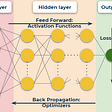## ROS for dummies. Part 3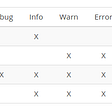## Data Science #12: Mini Project: Heart Disease Prediction using Machine Learning.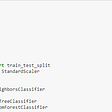## An Overview on MobileNet: An Efficient Mobile Vision CNN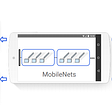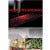Software Engineer for Big Data distributed systems

## CNN Feature Extraction: Between ReLU, Tanh and Sigmoid.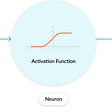## CNN — Right Approach Behind It## What’s next to improve the model accuracy of Convolutional Neural Networks (CNNs)## Indian Currency Classifier